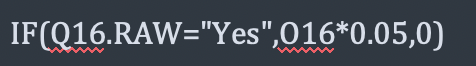# "IF Formula"

Require a formula to apply a Tax calculation based on vendor status.
EG, If a vendor is registered, a tax calculation (10%) is charged on the base price, if not registered no calculation to be applied.
Formula resides in G3 for the Tax amount.
IF((A3=“Yes”),(E1*.10))
Where "A3="Yes or “No”
E1= Invoice Amount

Simple task I know but just cant Ragic to emulate an excel formula.
Any help would be appreciated.

The syntax is a little different here.
`IF(A3=“Yes”, E1*0.10, 0)`

I like to consult the “List of Support Formulas” section of this document for syntax:

Hi,

IF(A3=“Yes”,E1*0.10,0)

Many thanks for your reply, I did check the formulas page but wasn’t able to locate this issue.
The formula is below, the system accepts the formula on the design page but doesn’t actually execute on data entry. Any ideas ?
IF(A3=“Yes”,E1*0.10,0)

Try
IF(A3.RAW=“Yes”,E1*0.10,0)

Works like a charm many many thanks 1. For the solution 2. For being SOOO responsive.
I am so glad I stumbled upon RAGIC.

hi Jit,

I try above method, but it’s still not working.
but if I use “1” instead of “no”, it works. pls kindly help me

to correct formula. thanks a lot.

Hi,